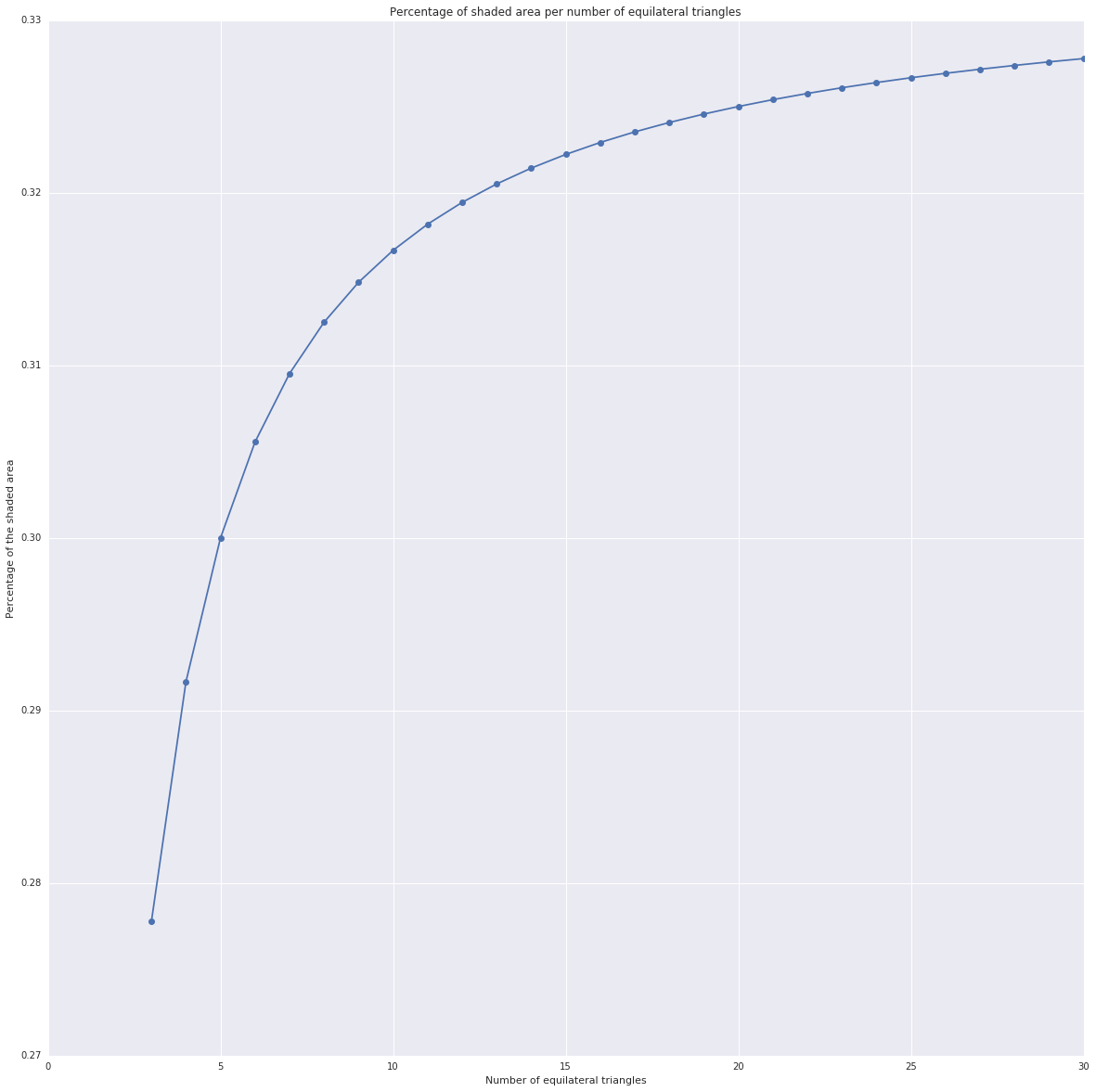# Triangle Fraction¶

A puzzle addressed during a MathsJam session (January 2016).Three equilateral triangles. Which fraction is shaded?

Found on Twitter, posted by @math8_teacher

Well, time for some geometry then. Hmm what about being lazy instead and let Python do the calculations? :)

In :
from sympy.geometry import Point, Segment, Triangle, intersection
from sympy import sqrt

import matplotlib.pyplot as plt
%matplotlib inline
import seaborn as sns

In :
def ratio(side):

# Height of an equilateral triangle
height = side * sqrt(3) / 2

# Points of the triangles on the base line
p0 = Point(0, 0)
p1 = Point(side, 0)
p2 = Point(2 * side, 0)
p3 = Point(3 * side, 0)

# Points of the triangles on the "top" line
p4 = Point(0.5 * (p1.x + p0.x), height)
p5 = Point(0.5 * (p2.x + p1.x), height)
p6 = Point(0.5 * (p3.x + p2.x), height)

# The 3 segments we are only interested in
# (that will intersect the last one)
s1 = Segment(p1, p4)
s2 = Segment(p1, p5)
s3 = Segment(p2, p5)

# The "big" segment that intersects everything
s4 = Segment(p0, p6)

# Intersections of the "big" segment with the others
i1 = intersection(s1, s4)
i2 = intersection(s2, s4)
i3 = intersection(s3, s4)

# The normal triangles
tri1 = Triangle(p0, p1, p4)
tri2 = Triangle(p1, p2, p5)
tri3 = Triangle(p2, p3, p6)

# The triangles constructed with the intersection points
tri4 = Triangle(p0, i1, p4)
tri5 = Triangle(i2, i3, p5)

res = (tri4.area + tri5.area) / (tri1.area + tri2.area + tri3.area)
return res

In :
# Calculate for 3 triangles of side 1
print('Ratio:', ratio(1))

Ratio: 5/18

In :
# The ratio should not depend on the side of the triangles
for i in range(2, 10):
assert ratio(i) == ratio(1)

print('All good!')

All good!


## Extension for 4, 5, and more triangles¶

The figure only shows 3 triangles. And for now the code does the calculation in a very manual way, defining the points, segments, triangles one by one.

Is it possible to rewrite the ratio function to be generic and handle the case for more triangles?

In :
def ratio_n(n, side):

# Height of an equilateral triangle
height = side * sqrt(3) / 2

ps = []
# Points of the triangles on the base line
for k in range(n + 1):
ps.append(Point(side * k, 0))

# Points of the triangles on the "top" line
for k in range(n):
ps.append(Point(0.5 * (ps[k+1].x + ps[k].x), height))

# The segments we are only interested in
segs = []
for k in range(1, n):
segs.append(Segment(ps[k], ps[k+n]))
segs.append(Segment(ps[k], ps[k+n+1]))

# The "big" segment that intersects everything
big = Segment(ps, ps[-1])

# The normal triangles
tris = []
for k in range(n):
tris.append(Triangle(ps[k], ps[k+1], ps[k+1+n]))

# The triangles constructed with the intersection points
for k in range(n-1):
if k == 0:
it = intersection(segs, big)
else:
kk = 2 * k
it1 = intersection(segs[kk], big)
it2 = intersection(segs[kk-1], big)

area_total = sum([abs(tri.area) for tri in tris])
return res


Let's first verify the function calcultes the same result found above for 3 triangles.

In :
print('Ratio for 3 triangles with the generic function:', ratio_n(3, 1))

Ratio for 3 triangles with the generic function: 5/18


Good. Now what happens when there are more triangles?

In :
SIDE = 10
LIMIT = 31  # Up to 30 triangles
ratios = []
xs = list(range(3, LIMIT))
for i in xs:
ratio_i = ratio_n(i, SIDE)
ratios.append(ratio_i)
print('Ratio for', i, 'triangles:', ratio_i, '~=', ratio_i.evalf())

Ratio for 3 triangles: 5/18 ~= 0.277777777777778
Ratio for 4 triangles: 7/24 ~= 0.291666666666667
Ratio for 5 triangles: 3/10 ~= 0.300000000000000
Ratio for 6 triangles: 11/36 ~= 0.305555555555556
Ratio for 7 triangles: 13/42 ~= 0.309523809523810
Ratio for 8 triangles: 5/16 ~= 0.312500000000000
Ratio for 9 triangles: 17/54 ~= 0.314814814814815
Ratio for 10 triangles: 19/60 ~= 0.316666666666667
Ratio for 11 triangles: 7/22 ~= 0.318181818181818
Ratio for 12 triangles: 23/72 ~= 0.319444444444444
Ratio for 13 triangles: 25/78 ~= 0.320512820512820
Ratio for 14 triangles: 9/28 ~= 0.321428571428571
Ratio for 15 triangles: 29/90 ~= 0.322222222222222
Ratio for 16 triangles: 31/96 ~= 0.322916666666667
Ratio for 17 triangles: 11/34 ~= 0.323529411764706
Ratio for 18 triangles: 35/108 ~= 0.324074074074074
Ratio for 19 triangles: 37/114 ~= 0.324561403508772
Ratio for 20 triangles: 13/40 ~= 0.325000000000000
Ratio for 21 triangles: 41/126 ~= 0.325396825396825
Ratio for 22 triangles: 43/132 ~= 0.325757575757576
Ratio for 23 triangles: 15/46 ~= 0.326086956521739
Ratio for 24 triangles: 47/144 ~= 0.326388888888889
Ratio for 25 triangles: 49/150 ~= 0.326666666666667
Ratio for 26 triangles: 17/52 ~= 0.326923076923077
Ratio for 27 triangles: 53/162 ~= 0.327160493827161
Ratio for 28 triangles: 55/168 ~= 0.327380952380952
Ratio for 29 triangles: 19/58 ~= 0.327586206896552
Ratio for 30 triangles: 59/180 ~= 0.327777777777778

In :
plt.figure(figsize=(20,20), dpi=80)
plt.plot(xs, ratios, 'o-')
plt.xlabel('Number of equilateral triangles')
plt.title('Percentage of shaded area per number of equilateral triangles')

Out:
<matplotlib.text.Text at 0x7f577bb172e8>## Observations¶

That's quite interesting! With some optimizations in the ratio_n function, we could calculate the ratio for more triangles.

But looking at the graph, we can ask at least two questions:

• Does the ratio converge to 1/3? (0.333333...)
• If yes, how to prove it?

I will let these two questions as an open challenge :)